JEE  >  Test: Meaning of Derivatives

# Test: Meaning of Derivatives

Test Description

## 10 Questions MCQ Test Mathematics For JEE | Test: Meaning of Derivatives

Test: Meaning of Derivatives for JEE 2022 is part of Mathematics For JEE preparation. The Test: Meaning of Derivatives questions and answers have been prepared according to the JEE exam syllabus.The Test: Meaning of Derivatives MCQs are made for JEE 2022 Exam. Find important definitions, questions, notes, meanings, examples, exercises, MCQs and online tests for Test: Meaning of Derivatives below.
Solutions of Test: Meaning of Derivatives questions in English are available as part of our Mathematics For JEE for JEE & Test: Meaning of Derivatives solutions in Hindi for Mathematics For JEE course. Download more important topics, notes, lectures and mock test series for JEE Exam by signing up for free. Attempt Test: Meaning of Derivatives | 10 questions in 10 minutes | Mock test for JEE preparation | Free important questions MCQ to study Mathematics For JEE for JEE Exam | Download free PDF with solutions
 1 Crore+ students have signed up on EduRev. Have you?
Test: Meaning of Derivatives - Question 1

###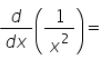Detailed Solution for Test: Meaning of Derivatives - Question 1

d/dx(1/x2 ) = -2 (x -2-1) = -2* (x-3) = -2/x3

Test: Meaning of Derivatives - Question 2

### The derivative of f(x) = 99x at x = 100 is:​

Detailed Solution for Test: Meaning of Derivatives - Question 2

f'(100) = lim(h→0) [f(100+h) - f(100)]/h
= lim(h→0) [99(100+h) - 99(100)]/h
= lim(h→0) [9900 + 99h - 9900]/h
= lim(h→0) 99h/h
= lim(h→0) 99
= 99

Test: Meaning of Derivatives - Question 3

### Find the derivative of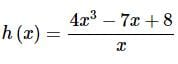Detailed Solution for Test: Meaning of Derivatives - Question 3

We’ll first need to divide the function out and simplify before we take the derivative. Here is the rewritten function.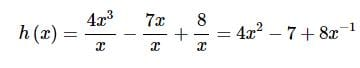The derivative is,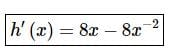Test: Meaning of Derivatives - Question 4

Derivative of sum of two functions is _____ of the derivatives of the functions.​

Detailed Solution for Test: Meaning of Derivatives - Question 4

In calculus, the sum rule in differentiation is a method of finding the derivative of a function that is the sum of two other functions for which derivatives exist. This is a part of the linearity of differentiation.

Test: Meaning of Derivatives - Question 5

The derivative of the constant function f(x) = a for a fixed real number ‘a’ is:​

Detailed Solution for Test: Meaning of Derivatives - Question 5

Let f(x) = a, where a is a fixed real number.
So, f(x+h) = a
So, d/dx(f(x)) = lim(h→0)f[(x+h)−f(x)]/h
= lim(h→0) (a−a)/h
= 0
Hence, d/dx(a) = 0 , where a is a fixed real number.

Test: Meaning of Derivatives - Question 6

Derivative of sum of two functions is sum of the derivatives of the functions. If , f and g be two functions such that their derivatives are defined in ______.​

Detailed Solution for Test: Meaning of Derivatives - Question 6
• The process of determining the derivative of a function is known as differentiation. It is clearly visible that the basic concept of derivative of a function is closely intertwined with limits. Therefore, it can be expected that the rules of derivatives are similar to that of limits.
• The following rules are a part of algebra of derivatives:
Consider f and g to be two real-valued functions such that the differentiation of these functions is defined in a common domain.
Test: Meaning of Derivatives - Question 7

The derivative of f(x) = 1/x3

Detailed Solution for Test: Meaning of Derivatives - Question 7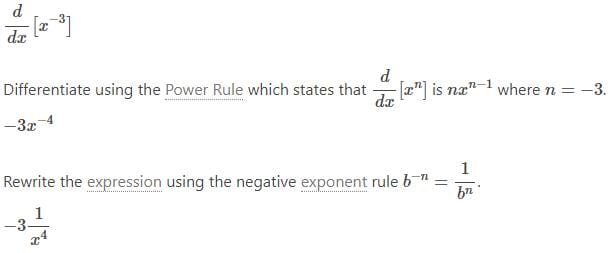Test: Meaning of Derivatives - Question 8

Derivative of quotient of two functions f(x) and g(x); g(x) ≠ 0 is given by  is given by

Detailed Solution for Test: Meaning of Derivatives - Question 8

This is a formula of question.

Test: Meaning of Derivatives - Question 9

If f is a real valued function and c is a point in its domain, then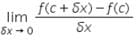is ;

Detailed Solution for Test: Meaning of Derivatives - Question 9

This is a formula for finding derivative or differentiation which is represented by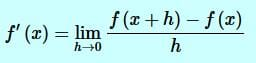but here at the place of x , c is written So this is equal to f'(c)

Test: Meaning of Derivatives - Question 10

The derivative at x = 2/3 of the function f(x) = 3x is:​

Detailed Solution for Test: Meaning of Derivatives - Question 10

f(x) = 3x
f’(x) = lim(h→0) [f(x+h) - f(x)]/h
= f(x+h) = 3(x+h)
f’(x) = = lim(h→0) [3(x+h) - 3(x)]/h
Putting x = ⅔
f’(⅔) = lim(h→0) [3(⅔+h) - 3(⅔)]/h
= lim(h→0) [(6+3h)-6]/h
=lim(h→0) 3h/h
lim(h→0) 3
The derivative of the function f’(⅔) = 3

## Mathematics For JEE

125 videos|301 docs|299 tests
 Use Code STAYHOME200 and get INR 200 additional OFF Use Coupon Code
Information about Test: Meaning of Derivatives Page
In this test you can find the Exam questions for Test: Meaning of Derivatives solved & explained in the simplest way possible. Besides giving Questions and answers for Test: Meaning of Derivatives, EduRev gives you an ample number of Online tests for practice

## Mathematics For JEE

125 videos|301 docs|299 tests